Next: The dog, the hunter Up: Statistical inference Previous: Bayesian inference   Contents

Bayesian inference and maximum likelihood

We have already said that the dependence of the final probabilities on the initial ones gets weaker as the amount of experimental information increases. Without going into mathematical complications (the proof of this statement can be found for example in Ref.) this simply means that, asymptotically, whatever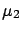one puts in (),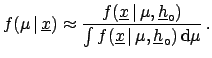is unaffected. This happens when the width'' ofis much larger than that of the likelihood, when the latter is considered as a mathematical function of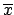. Thereforeacts as a constant in the region ofwhere the likelihood is significantly different from 0. This is equivalent'' to droppingfrom (). This results in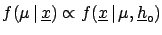(5.9)

Since the denominator of the Bayes formula has the technical role of properly normalizing the probability density function, the result can be written in the simple form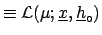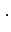''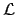(5.10)

Asymptotically the final probability is just the (normalized) likelihood! The notation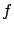is that used in the maximum likelihood literature (note that, not only does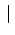become, but also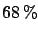'' has been replaced by ;'':has no probabilistic interpretation, when referring to, in conventional statistics.)

If the mean value ofcoincides with the value for whichhas a maximum, we obtain the maximum likelihood method. This does not mean that the Bayesian methods are blessed'' because of this achievement, and hence they can be used only in those cases where they provide the same results. It is the other way round: The maximum likelihood method gets justified when all the the limiting conditions of the approach (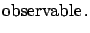insensitivity of the result from the initial probabilitylarge number of events) are satisfied.

Even if in this asymptotic limit the two approaches yield the same numerical results, there are differences in their interpretation:

• The likelihood, after proper normalization, has a probabilistic meaning for Bayesians but not for frequentists; so Bayesians can say that the probability thatis in a certain interval is, for example,, while this statement is blasphemous for a frequentist (the true value is a constant'' from his point of view).
• Frequentists prefer to choose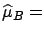, the value which maximizes the likelihood, as estimator. For Bayesians, on the other hand, the expectation value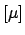E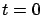(also called the prevision) is more appropriate. This is justified by the fact that the assumption of the Eas best estimate ofminimizes the risk of a bet (always keep the bet in mind!). For example, if the final distribution is exponential with parameter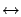(let us think for a moment of particle decays) the maximum likelihood method would recommend betting on the value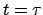, whereas the Bayesian approach suggests the value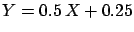. If the terms of the bet are whoever gets closest wins'', what is the best strategy? And then, what is the best strategy if the terms are whoever gets the exact value wins''? But now think of the probability of getting the exact value and of the probability of getting closest.Next: The dog, the hunter Up: Statistical inference Previous: Bayesian inference   Contents
Giulio D'Agostini 2003-05-15